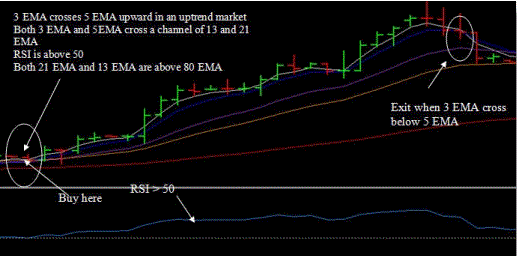# EMA Trend with RSI

EMA Trend with RSI is a trend following strategy profittable that use only two indicators: EMA and RSI. This is a swing trading system.
Currency pairs: USD/JPY, GBP/USD, EUR/USD, EURGBP, USD/CHF, AUD/USD, GBP/JPY.
Time Frame 30 min or higher.
Forex Indicators:
3 Exponential moving average, close;
5 Exponential moving average, close.
13 Exponential moving average, close.
21 Exponential moving average, close.
80 Exponential moving average, close.
3 Exponential moving average, close.
RSI period 21.
80 Exponential moving average, close, indicates trend direction.
When the price goes above 80 EMA, there is an uptrend,
when the price goes below 80 EMA, there is an downtrend,
21 EMA and 13 EMA indicates the short trend direction.
If 13 EMA is above 21 EMA  there is an uptrend
If 13 EMA is below 21 EMA  there is an downtrend
Also RSI (21) value indicates the trend is above 50 level  an uptrend, below downtrend.
3 Exponential moving average  crosses 5 EMA upward.
3 EMA and 5EMA cross above 13 and 21 EMA.
RSI is above 50.
13 EMA and 21 EMA cross above 80 EMA.
when 3 EMA crosses below 5 EMA or RSI < 50 level.
Sell
3 Exponential moving average  crosses 5 EMA downward.
3 EMA and 5EMA cross above 13 and 21 EMA.
RSI is below 50 level.
13 EMA and 21 EMA cross below 80 EMA.
Exit Sell
when 3 EMA crosses above 5 EMA or RSI > 50 level.
Stop loss
For 30 min time frame stop loss = 16 – 25 pips for 60 min time frame 20 -30 pips depending bythe volatility of the market.
Buy Example: EUR/USD 1 hour timeframe
We entered when 3 EMA crosses 5 EMA upward in an uptrend market. Both 3 EMA and 5EMA cross a channel of 13 and 21 EMA. RSI is above 50. Both 21 EMA and 13 EMA are above 80 EMA. And we closed the trade when 3 EMA cross below 5 EMA.
Example 2: EUR/USD 4-hour timeframe

We entered when 3 EMA crosses 5 EMA upward in an uptrend market. Both 3 EMA and
5EMA cross a channel of 13 and 21 EMA. RSI is above 50. Both 21 EMA and 13 EMA are above 80 EMA. And we closed the trade when 3 EMA cross below 5 EMA.
Example 3: EUR/USD 4-hour timeframeWe entered when 3 EMA crosses 5 EMA upward in an uptrend market. Both 3 EMA and 5EMA cross a channel of 13 and 21 EMA. RSI is above 50. Both 21 EMA and 13 EMA are above 80 EMA.
And we closed the trade when 3 EMA cross below 5 EMA.
Both 21 EMA and 13 EMA are above 80 EMA.
Both 3 EMA and 5EMA cross a channel of 13 and 21 EMA.
RSI is above 50 Both 21 EMA and 13 EMA are above 80 EMA.
RSI is above 50 Both 21 EMA and 13 EMA are above 80 EMA.
Example 4: EUR/USD 4-hour timeframe:

We entered a short trade when 3 EMA crosses 5 EMA downward in an downtrend
market. Both 3 EMA and 5EMA cross a channel of 13 and 21 EMA. RSI is below 50.
Both 21 EMA and 13 EMA are below 80 EMA.
We closed this short trade when 3 EMA cross above 5 EMA.
Example 5: EUR/USD 1-hour timeframe:
We entered a short trade when 3 EMA crosses 5 EMA downward in an downtrend
market. Both 3 EMA and 5EMA cross a channel of 13 and 21 EMA. RSI is below 50.
Both 21 EMA and 13 EMA are below 80 EMA.
We closed this short trade when 3 EMA cross above 5 EMA.
Example 6: EUR/USD daily timeframe:
We entered a short trade when 3 EMA crosses 5 EMA downward in an downtrend
market. Both 3 EMA and 5EMA cross a channel of 13 and 21 EMA. RSI is below 50.
Both 21 EMA and 13 EMA are below 80 EMA.
We closed this short trade when 3 EMA cross above 5 EMA.
```

```